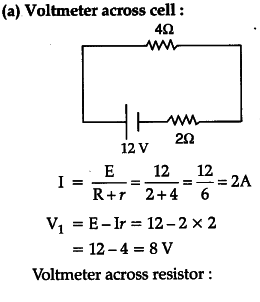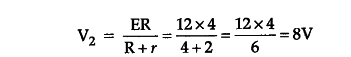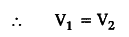# A battery of emf 12V and internal resistance 2 Q is connected to a 4 Q resistor as shown in the figure

A battery of emf 12V and internal resistance 2 Q is connected to a 4 Q resistor as shown in the figure.
(a) Show that a voltmeter when placed across the cell and across the resistor, in turn, gives the same reading.
(b) To record the voltage and the current in the circuit,
why is voltmeter placed in parallel and ammeter in series in the circuit ?b) Voltmeter connects in parallel because it measures the difference in voltage potential between two points. Ammeter connects in series because it needs to measure the total amount of current flow.